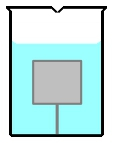Start typing, then use the up and down arrows to select an option from the list.

# A wooden cube, 1 m on all sides and having density 800 kg/m3 , is held under water in a large container by a string, as shown below. The top of the cube is exactly 2 m below the water line. Calculate the difference between the force applied by water to the top and to the bottom faces of the cube (Hint:calculate the two forces, then subtract. Use g=10 m/s2.)### Watch next

Master Pressure and Atmospheric Pressure with a bite sized video explanation from Patrick Ford

Start learning